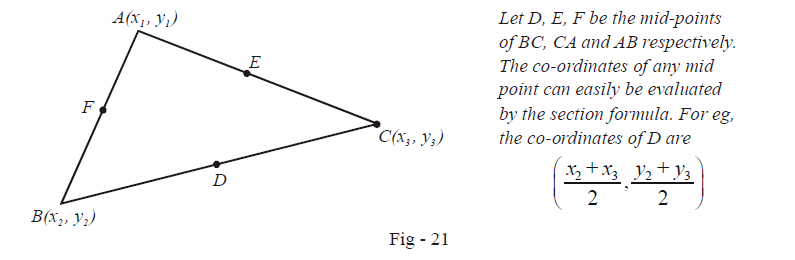# Examples on Concurrency of Three Lines Set 1

Go back to  'Straight Lines'

Example – 9

Prove that the three lines L1, L2 and L3 whose equations have been mentioned in the preceeding discussion, are concurrent if we can find three constants  $${{\lambda }_{1}},{{\lambda }_{2}}\,\,\text{and}\,\,{{\lambda }_{3}}$$ such that

${{\lambda }_{1}}{{L}_{1}}\,\,+\,\,{{\lambda }_{2}}{{L}_{2}}+{{\lambda }_{3}}{{L}_{3}}=0$

Solution: Assume that L1 and L2 intersect at the point P whose co-ordinates are (x0, y0) P should satisfy the equations of both Land L2.

\begin{align} & {{L}_{1}}\left( \text{at}\,P \right)\equiv {{a}_{1}}{{x}_{0}}+{{b}_{1}}{{y}_{0}}+{{c}_{1}}=0\qquad \qquad ...\text{ }\left( 1 \right) \\ & {{L}_{2}}\left( \text{at}\,P \right)\equiv {{a}_{2}}{{x}_{0}}+{{b}_{2}}{{y}_{0}}+{{c}_{2}}=0\qquad \qquad ...\text{ }\left( 2 \right) \\ \end{align}

Now assume that we can find three non-zero constants $${{\lambda }_{1}},{{\lambda }_{2}}\,\,\text{and}\,\,{{\lambda }_{3}}$$ such that $${{\lambda }_{1}}{{L}_{1}}+{{\lambda }_{2}}{{L}_{2}}+{{\lambda }_{3}}{{L}_{3}}=0$$  . We will prove that due to this condition, L3 will definitely have to pass through P:

\begin{align} &\qquad\quad {{\lambda }_{1}}{{L}_{1}}+{{\lambda }_{2}}{{L}_{2}}+{{\lambda }_{3}}{{L}_{3}}=0 \\ & \Rightarrow\quad {{L}_{3}}=\left( -\frac{{{\lambda }_{1}}}{{{\lambda }_{3}}} \right){{L}_{1}}+\left( -\frac{{{\lambda }_{2}}}{{{\lambda }_{3}}} \right){{L}_{2}} \end{align}

If we evaluate the value of L3 at P, we get

\begin{align} & {{L}_{3}}\left( \text{at}\,P \right)=\left( -\frac{{{\lambda }_{1}}}{{{\lambda }_{3}}} \right)\times {{L}_{1}}\left( \text{at}\,P \right)+\left( -\frac{{{\lambda }_{2}}}{{{\lambda }_{3}}} \right)\times {{L}_{2}}\left( \text{at}\,P \right) \\ & \qquad\qquad=\left( -\frac{{{\lambda }_{1}}}{{{\lambda }_{3}}} \right)\times 0+\left( -\frac{{{\lambda }_{2}}}{{{\lambda }_{3}}} \right)\times 0\qquad \qquad \left\{ \begin{gathered} \text{By}\,\,\,\left( 1 \right) \\ \text{and}\,\,\left( 2 \right) \end{gathered} \right\} \\ &\qquad\qquad =0 \end{align}

Since the value of L3 is 0 at P, the line Lmust pass through P. Thus, L1, L2 and L3 are concurrent.

Example – 10

Show that the medians of a triangle are concurrent.

Solution: Let the triangle have the vertices  $$A\left( {{x}_{1}},{{y}_{1}} \right),B\left( {{x}_{2}},{{y}_{2}} \right)\,\,\text{and}\,\,C\left( {{x}_{3}},{{y}_{3}} \right)$$ as in the figure below:From the two-point form of the equation of a line, we can write down the equations of AD, BE and CF.

The equation L1 of the median AD is:

\begin{align} & \qquad \quad\,\,\,\,{{L}_{1}}\equiv \frac{y-{{y}_{1}}}{x-{{x}_{1}}}=\frac{{{y}_{1}}-\begin{align}\frac{{{y}_{2}}+{{y}_{3}}}{2}\end{align}}{{{x}_{1}}-\begin{align}\frac{{{x}_{2}}+{{x}_{3}}}{2}\end{align}} \\ & \Rightarrow \qquad {{L}_{1}}\equiv \left( 2{{y}_{1}}-\left( {{y}_{2}}+{{y}_{3}} \right) \right)x-\left( 2{{x}_{1}}-\left( {{x}_{2}}+{{x}_{3}} \right) \right)y={{x}_{1}}\left( 2{{y}_{1}}-\left( {{y}_{2}}+{{y}_{3}} \right) \right)-{{y}_{1}}\left( 2{{x}_{1}}-\left( {{x}_{2}}+{{x}_{3}} \right) \right) \end{align}

By symmetry, we can write down the corresponding equations L2 and L3 of the medians BE and CF.

Observe carefully that when we subsequently add the three equations L1, L2 and L3, their left hand sides sum to 0. Thus, we have found three constants 1, 1 and 1 such that

\begin{align} & \qquad\quad \;1\cdot {{L}_{1}}+1\cdot {{L}_{2}}+1\cdot {{L}_{3}}=0 \\ & \Rightarrow \qquad {{L}_{1}},{{L}_{2}}\,\,\text{and}\,{{L}_{3}} \\ & \Rightarrow \qquad \text{The medians of any triangle are concurrent.} \end{align}